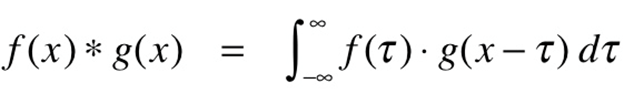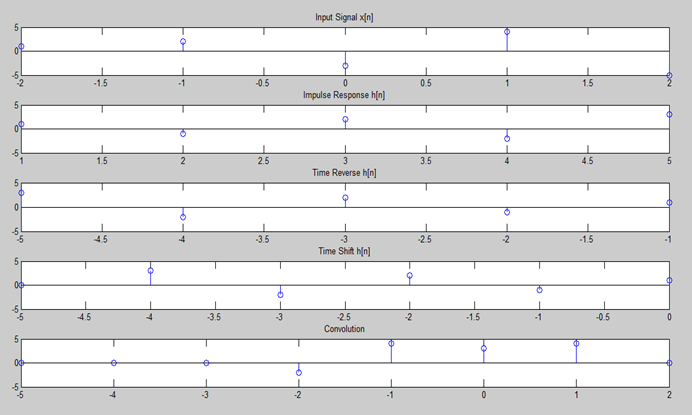# Convolution without Function in MATLAB

The convolution of two signals in the time domain is equivalent to the multiplication of their representation in frequency domain. Mathematically, we can write the convolution of two signals asAs everyone knows that in MATLAB there is a built-in conv(x, y) function, through which you can easily convolve two functions and calculate their convolution. But ever wonder how to find convolution in MATLAB without a conv function. Yes, you can manually convolve two functions by taking input signal as x[n] and impulse response as h[n] in MATLAB, and calculate their convolution respectively. For this you need to write the complete code of convolution process in order to manually perform convolution. To perform and do Convolution without function in MATLAB, simply open and create a new Script (.m file) in Matlab.

Step 1:

Open MATLAB << Then on the top left corner click on the File option << Click New << Then click Script.

Step 2:

Now write the following Matlab code given below of CONVOLUTION into the new script (.m file) and save the file in the Matlab directory with any name you want.

CONVOLUTION MATLAB CODE:

n11 = [-2:2];

x11 = [1 2 -3 4 -5];

n2 = [1:5];

x2 = [1 -1 2 -2 3];

d = abs(n11(end)-n2(1));

s = [ ];

a = [ ];

subplot(5,1,1)

stem(n11,x11)

title(‘Input Signal x[n]’)

subplot(5,1,2)

stem(n2,x2)

title(‘Impulse Response h[n]’)

[y n1] = sigfold(x2,n2)

subplot(5,1,3)

stem(n1,y)

title(‘Time Reverse h[n]’)

[n1,x1] = sigshift(y,n1,d)

subplot(5,1,4)

stem(n1,x1)

title(‘Time Shift h[n]’)

for i=1:length(x11)+length(n11-1)-1

[n12,x12] = sigshift(x1,n1,d)

[y23 n23] = sigmult(x11,n11,x12,n12)

subplot(5,1,5)

stem(n23,y23)

title(‘Convolution’)

end

Step 3:

Now Run the code, after you have saved the file.

OUTPUT:

n1 =

-5    -4    -3    -2    -1

y =

3    -2     2    -1     1

y =

3    -2     2    -1     1

n1 =

-5    -4    -3    -2    -1

n1 =

-5    -4    -3    -2    -1     0

x1 =

0     3    -2     2    -1     1

n12 =

-5    -4    -3    -2    -1     0     1

x12 =

0     0     3    -2     2    -1     1

y23 =

0     0     0    -2     4     3     4     0

n23 =

-5    -4    -3    -2    -1     0     1     2

n12 =

-5    -4    -3    -2    -1     0     1

x12 =

0     0     3    -2     2    -1     1

y23 =

0     0     0    -2     4     3     4     0

n23 =

-5    -4    -3    -2    -1     0     1     2

n12 =

-5    -4    -3    -2    -1     0     1

x12 =

0     0     3    -2     2    -1     1

y23 =

0     0     0    -2     4     3     4     0

n23 =

-5    -4    -3    -2    -1     0     1     2

n12 =

-5    -4    -3    -2    -1     0     1

x12 =

0     0     3    -2     2    -1     1

y23 =

0     0     0    -2     4     3     4     0

n23 =

-5    -4    -3    -2    -1     0     1     2

n12 =

-5    -4    -3    -2    -1     0     1

x12 =

0     0     3    -2     2    -1     1

y23 =

0     0     0    -2     4     3     4     0

n23 =

-5    -4    -3    -2    -1     0     1     2

n12 =

-5    -4    -3    -2    -1     0     1

x12 =

0     0     3    -2     2    -1     1

y23 =

0     0     0    -2     4     3     4     0

n23 =

-5    -4    -3    -2    -1     0     1     2

n12 =

-5    -4    -3    -2    -1     0     1

x12 =

0     0     3    -2     2    -1     1

y23 =

0     0     0    -2     4     3     4     0

n23 =

-5    -4    -3    -2    -1     0     1     2

n12 =

-5    -4    -3    -2    -1     0     1

x12 =

0     0     3    -2     2    -1     1

y23 =

0     0     0    -2     4     3     4     0

n23 =

-5    -4    -3    -2    -1     0     1     2

n12 =

-5    -4    -3    -2    -1     0     1

x12 =

0     0     3    -2     2    -1     1

y23 =

0     0     0    -2     4     3     4     0

n23 =

-5    -4    -3    -2    -1     0     1     2

FIGURE:This is how you can perform convolution and convolve two signals without a conv function in MATLAB. Hope you like this article. If you want to learn more about MATLAB you can read and view all the blog posts related to MALTAB here. MATLAB Learning

If you need any help and assistance, please comment down below so that we can help you. Good Luck!!

See More…